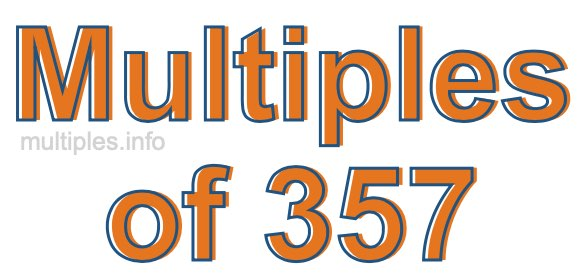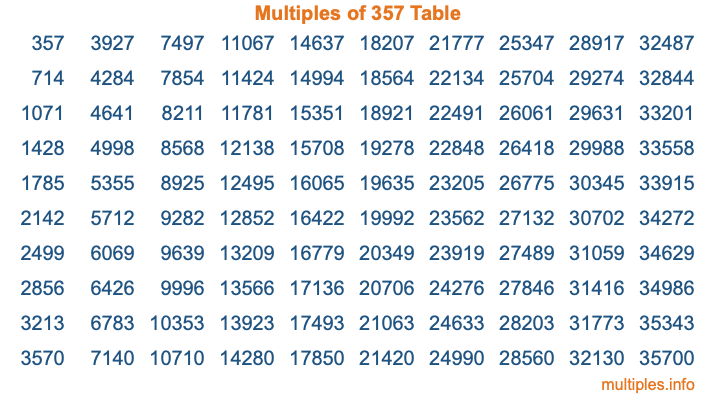Multiples of 357Welcome to the Multiples of 357 page. Here we will first teach you everything you will ever need to know about the multiples of 357, and then give you a study guide summary of everything we taught you to make sure you remember it all. Use this page to look up facts and learn information about the multiples of 357. This page will make you a multiples of three hundred fifty-seven expert!

Definition of Multiples of 357
Multiples of 357 are all the numbers that when divided by 357 equal an integer. Each of the multiples of 357 are called a multiple. A multiple of 357 is created by multiplying 357 by an integer.

Therefore, to create a list of multiples of 357, you start with 1 multiplied by 357, then 2 multiplied by 357, then 3 multiplied by 357, and so on for as long as you want. Thus, the list of the first five multiples of 357 is 357, 714, 1071, 1428, and 1785. To see a larger list of multiples of 357, see the printable image of Multiples of 357 further down on this page. We also have a category where you can choose any nth multiple of 357.

Multiples of 357 Checker
The Multiples of 357 Checker below checks to see if any number of your choice is a multiple of 357. In other words, it checks to see if there is any number (integer) that when multiplied by 357 will equal your number. To do that, we divide your number by 357. If the the quotient is an integer, then your number is a multiple of 357.

Is  a multiple of 357?

Least Common Multiple of 357 and ...
A Least Common Multiple (LCM) is the lowest multiple that two or more numbers have in common. This is also called the smallest common multiple or lowest common multiple and is useful to know when you are adding our subtracting fractions. Enter one or more numbers below (357 is already entered) to find the LCM.

Check out our LCM Calculator if you need more details about the Least Common Multiple or if you need the LCM for different numbers for adding and subtraction fractions.

nth Multiple of 357
As we stated above, 357 is the first multiple of 357, 714 is the second multiple of 357, 1071 is the third multiple of 357, and so on. Enter a number below to find the nth multiple of 357.

th multiple of 357

Multiples of 357 vs Factors of 357
357 is a multiple of 357 and a factor of 357, but that is where the similarities end. All postive multiples of 357 are 357 or greater than 357. All positive factors of 357 are 357 or less than 357.

Below is the beginning list of multiples of 357 and the factors of 357 so you can compare:

Multiples of 357: 357, 714, 1071, 1428, 1785, etc.

Factors of 357: 1, 3, 7, 17, 21, 51, 119, 357

As you can see, the multiples of 357 are all the numbers that you can divide by 357 to get a whole number. The factors of 357, on the other hand, are all the whole numbers that you can multiply by another whole number to get 357.

It's also interesting to note that if a number (x) is a factor of 357, then 357 will also be a multiple of that number (x).

Multiples of 357 vs Divisors of 357
The divisors of 357 are all the integers that 357 can be divided by evenly. Below is a list of the divisors of 357.

Divisors of 357: 1, 3, 7, 17, 21, 51, 119, 357

The interesting thing to note here is that if you take any multiple of 357 and divide it by a divisor of 357, you will see that the quotient is an integer.

Multiples of 357 Table
Below is an image of the first 100 multiples of 357 in a table. The table is in chronological order, column by column. The first column has the first ten multiples of 357, the second column has the next ten multiples of 357, and so on.The Multiples of 357 Table is also referred to as the 357 Times Table or Times Table of 357. You are welcome to print out our table for your studies.

Negative Multiples of 357
Although not often discussed or needed in math, it is worth mentioning that you can make a list of negative multiples of 357 by multiplying 357 by -1, then by -2, then by -3, and so on, to get the following list of negative multiples of 357:

-357, -714, -1071, -1428, -1785, etc.

Multiples of 357 Summary
Below is a summary of important Multiples of 357 facts that we have discussed on this page. To retain the knowledge on this page, we recommend that you read through the summary and explain to yourself or a study partner why they hold true.

There are an infinite number of multiples of 357.

A multiple of 357 divided by 357 will equal a whole number.

357 divided by a factor of 357 equals a divisor of 357.

The nth multiple of 357 is n times 357.

The largest factor of 357 is equal to the first positive multiple of 357.

357 is a multiple of every factor of 357.

357 is a multiple of 357.

A multiple of 357 divided by a divisor of 357 equals an integer.

357 divided by a divisor of 357 equals a factor of 357.

Any integer times 357 will equal a multiple of 357.

Multiples of a Number
Here you can get the multiples of another number, all with the same attention to detail as we did for multiples of 357 on this page.

Multiples of
Multiples of 358
Did you find our page about multiples of three hundred fifty-seven educational? Do you want more knowledge? Check out the multiples of the next number on our list!

Copyright  |   Privacy Policy  |   Disclaimer  |   Contact